# NCERT Solutions for Class 10 Maths Chapter 6 Triangles

NCERT Solutions for Class 10 Maths Chapter 6 Triangles

Students can use the link provided here to access and download the NCERT Solutions for Class 10 Maths Chapter 6, Triangles. The questions in Exercise 6.1 have been solved with great attention by our math specialists. This chapter guides students through the fundamental principles of triangles, as well as several approaches for proving the resemblance of triangles and other concepts.

NCERT Solutions for Class 10 Maths Chapter 6 Triangles | Free PDF Download Part 1

NCERT Solutions for Class 10 Maths Chapter 6 Triangles | Free PDF Download Part 2

When students solve the questions in the NCERT textbook, they follow the NCERT syllabus and norms, and tackling these questions over and over will help them acquire confidence. As a result, students who want to do well in their Maths exams in the first and second terms should do so.

The basic ideas of triangle are covered in NCERT Class 10 Maths Chapter 6, Triangles. We can state that the exercise is mostly concerned with brushing up on principles learned in earlier lessons. It is the first task in the chapter, and it focuses on the essential explanations. The following are some of the important things that are covered in chapter 6.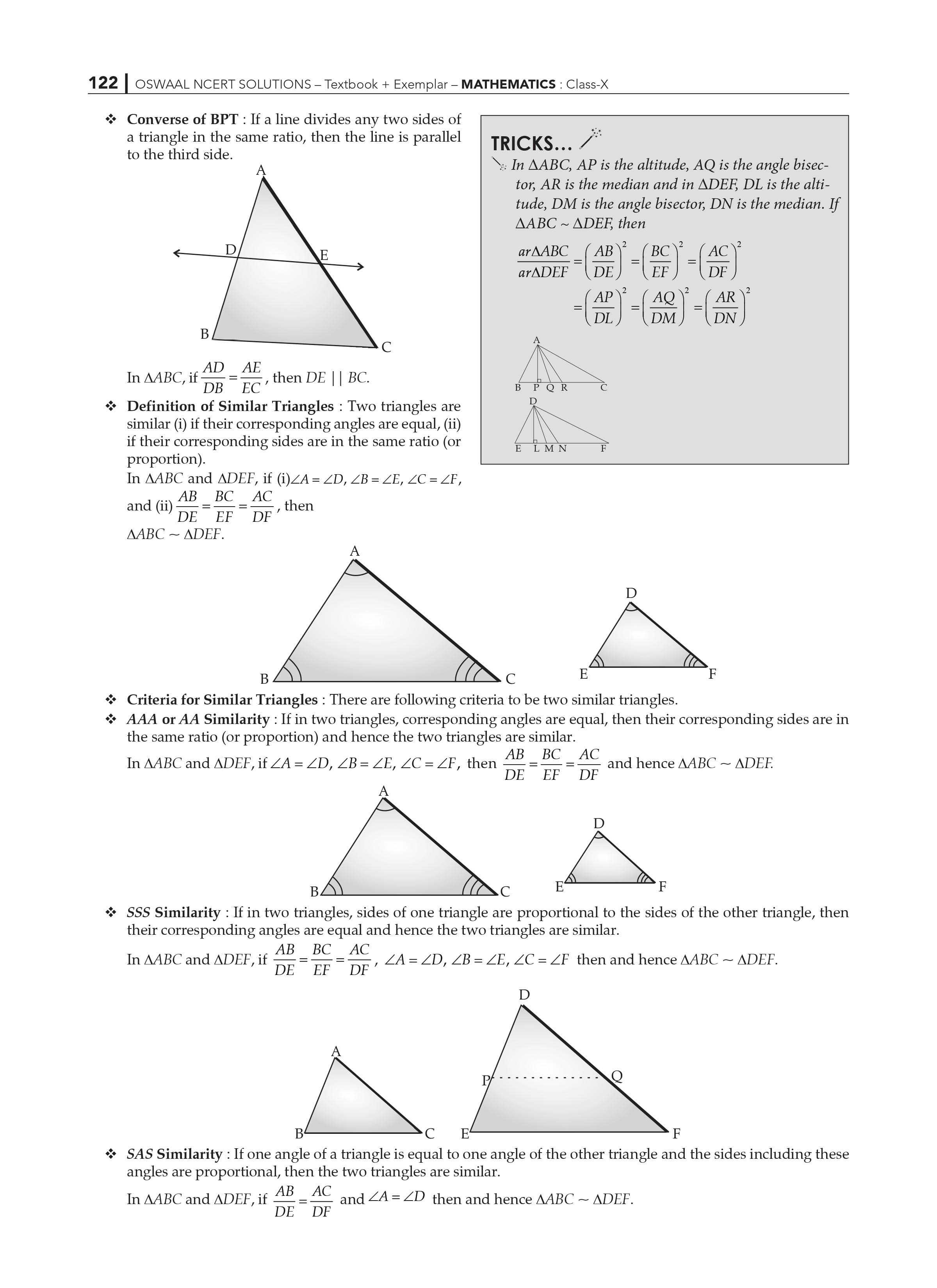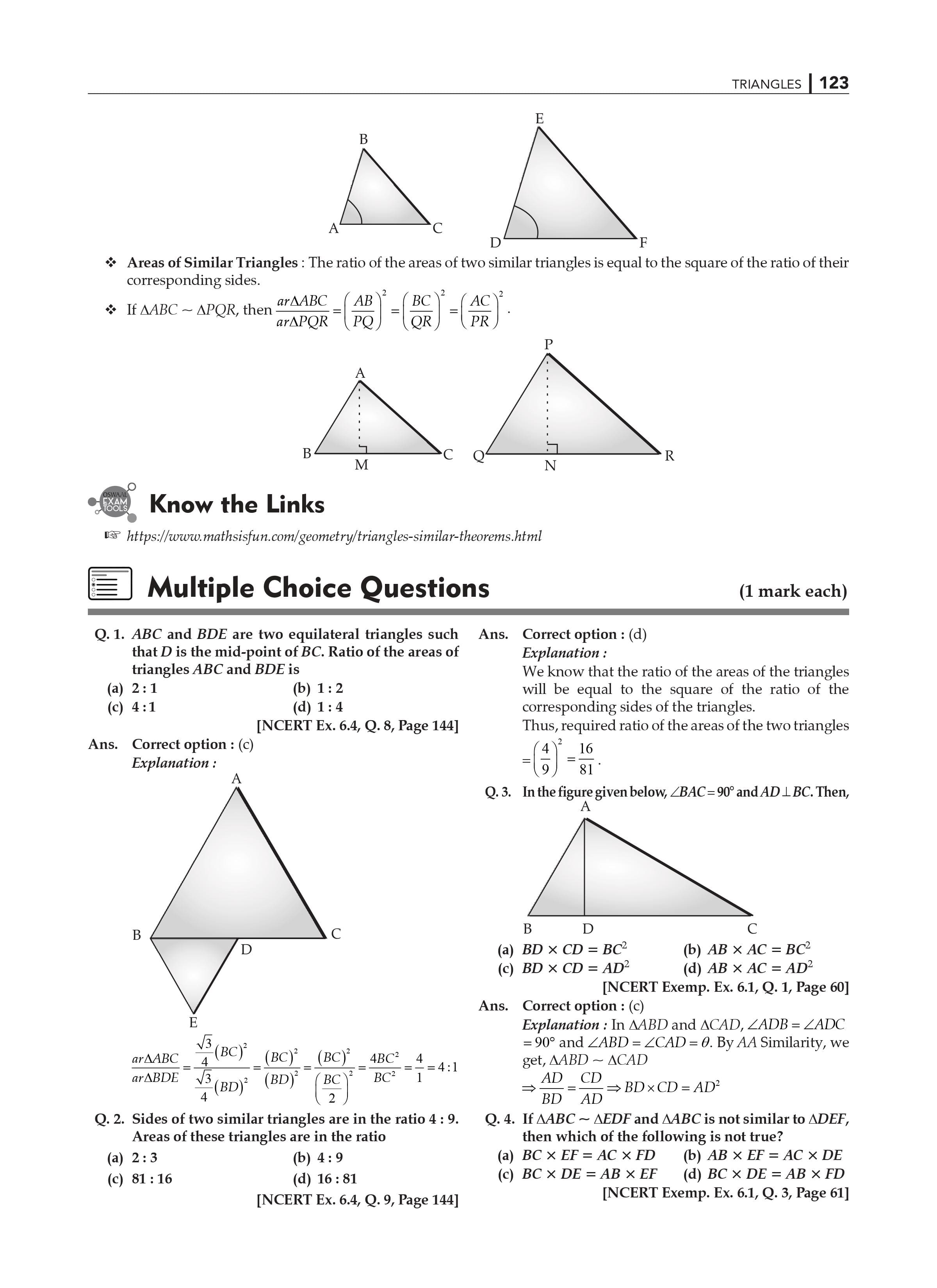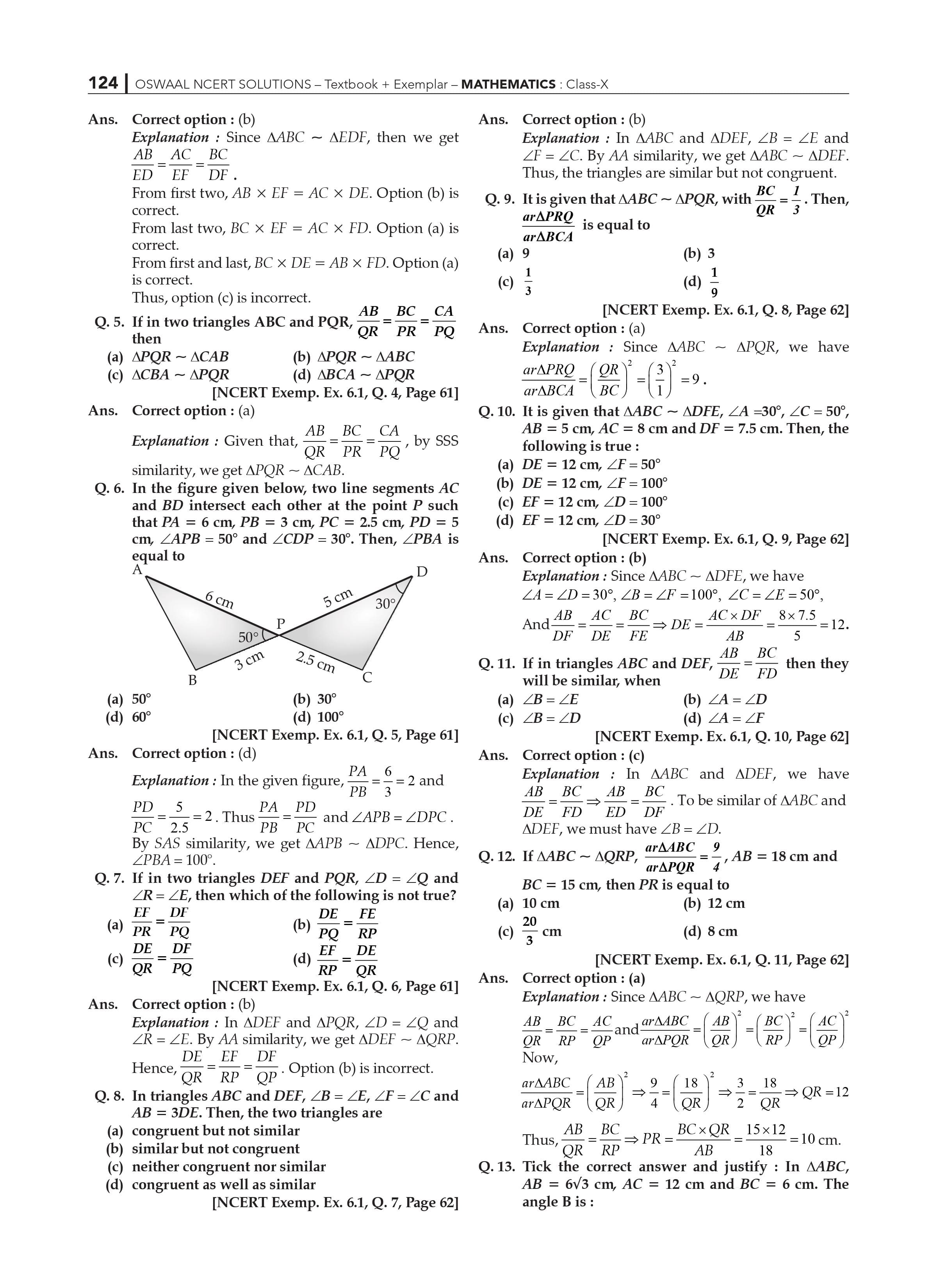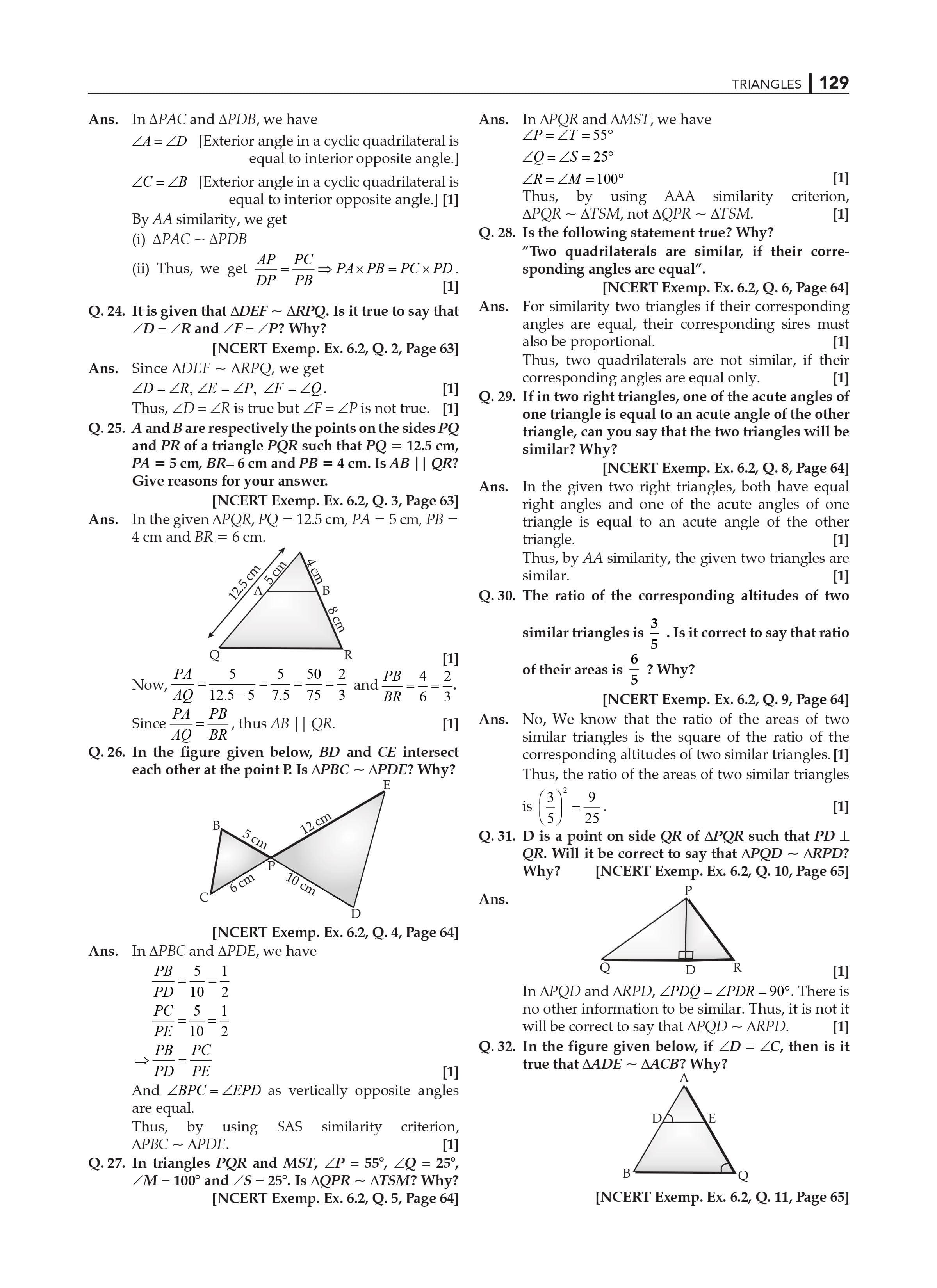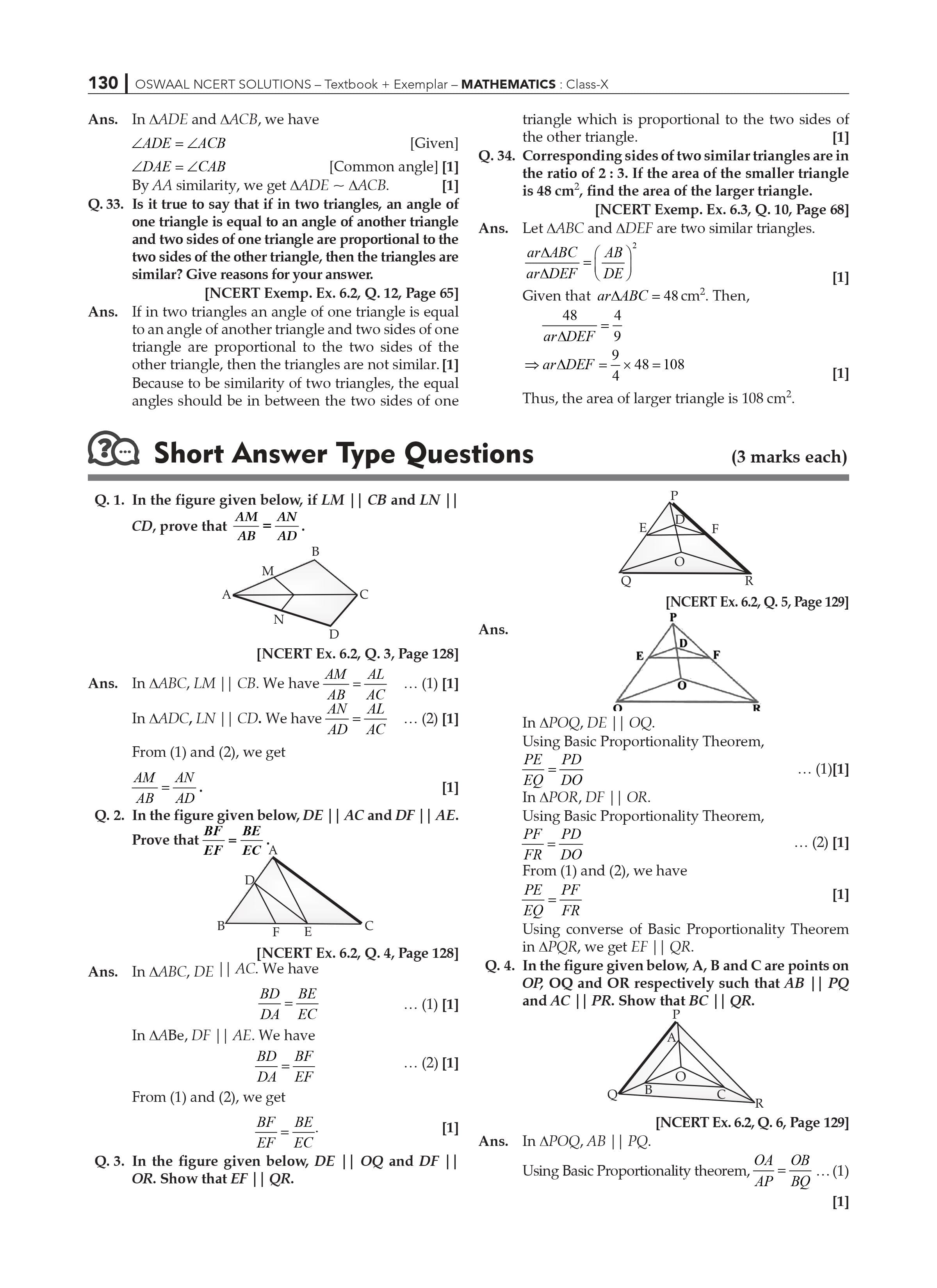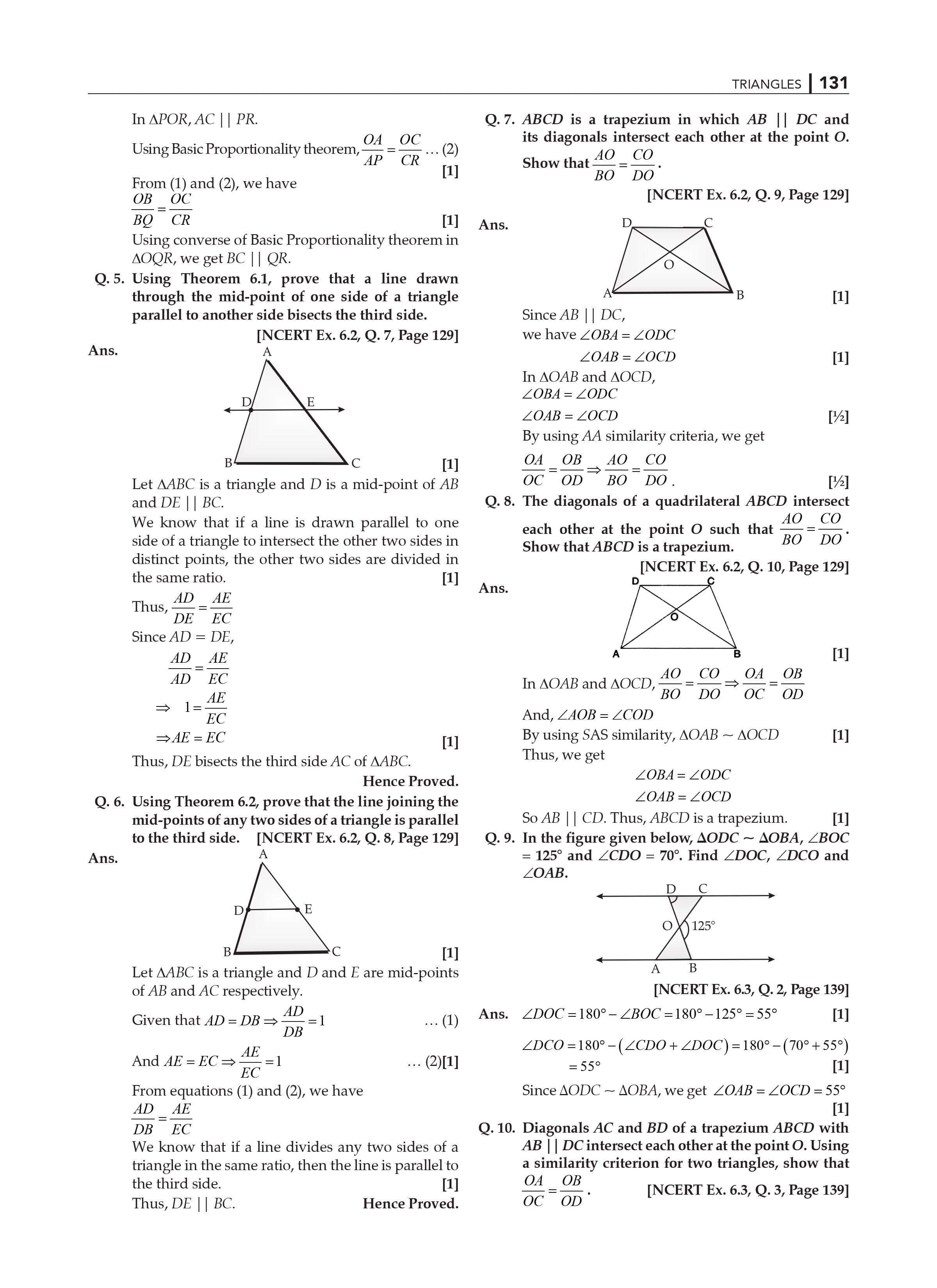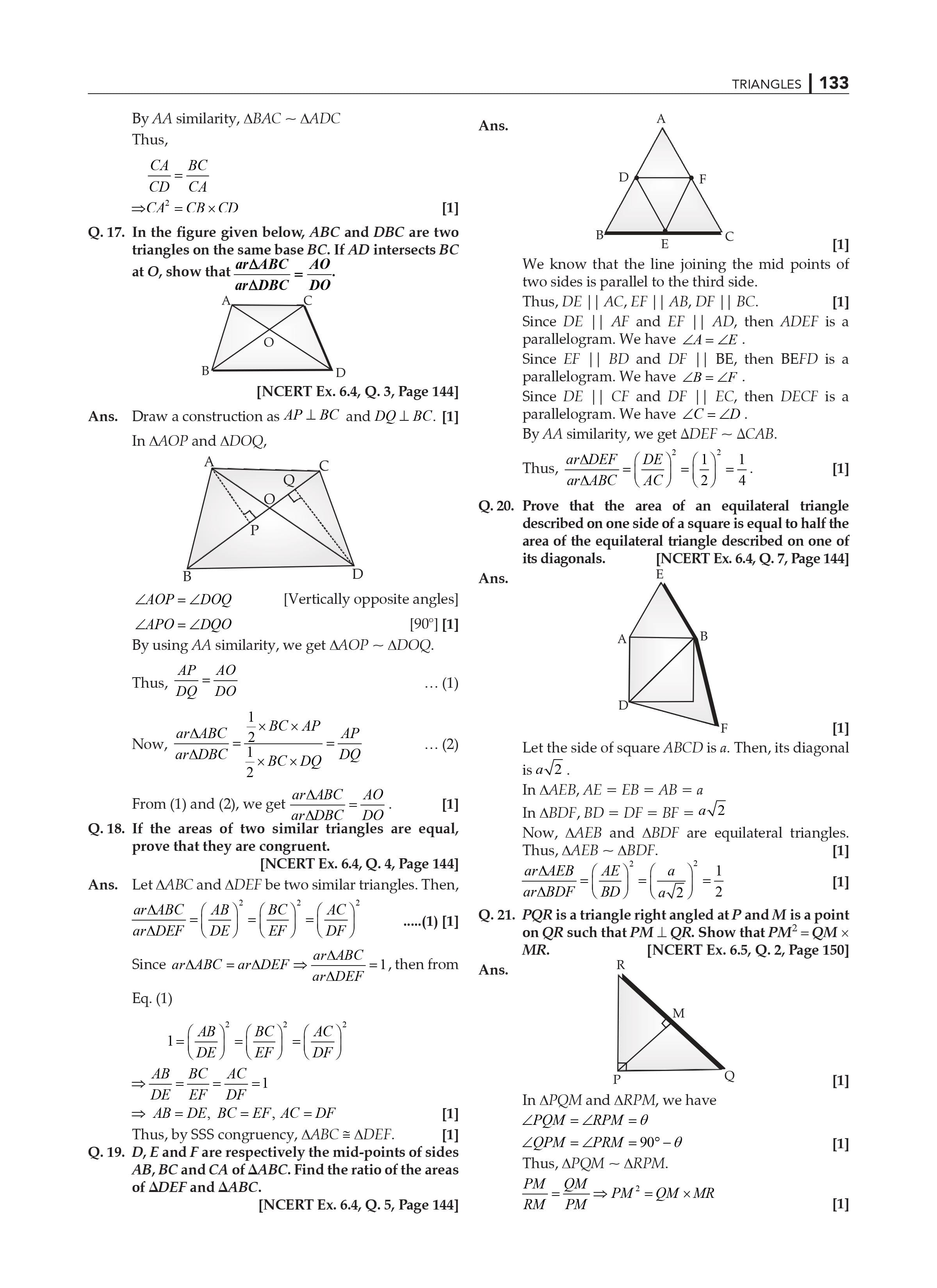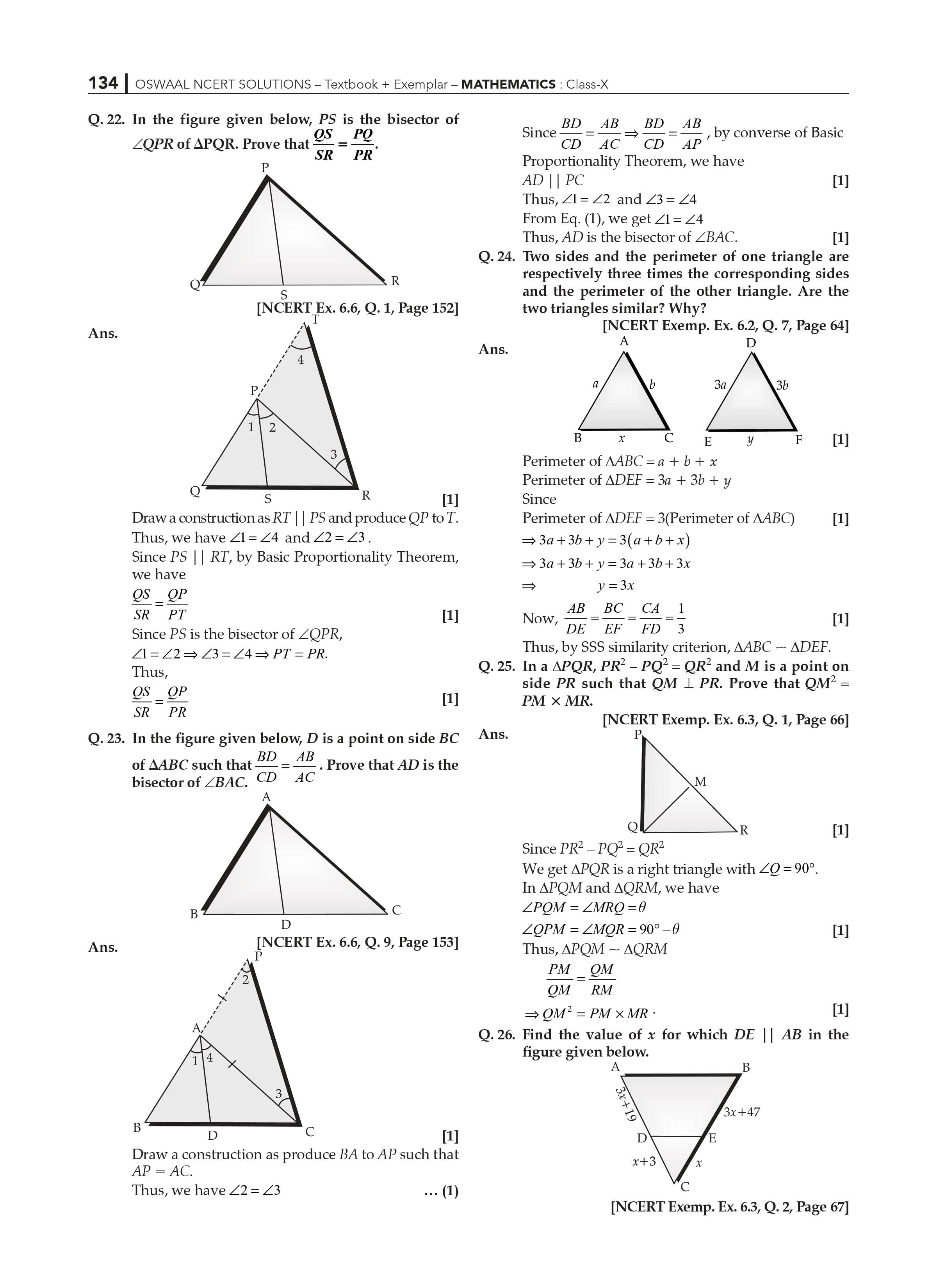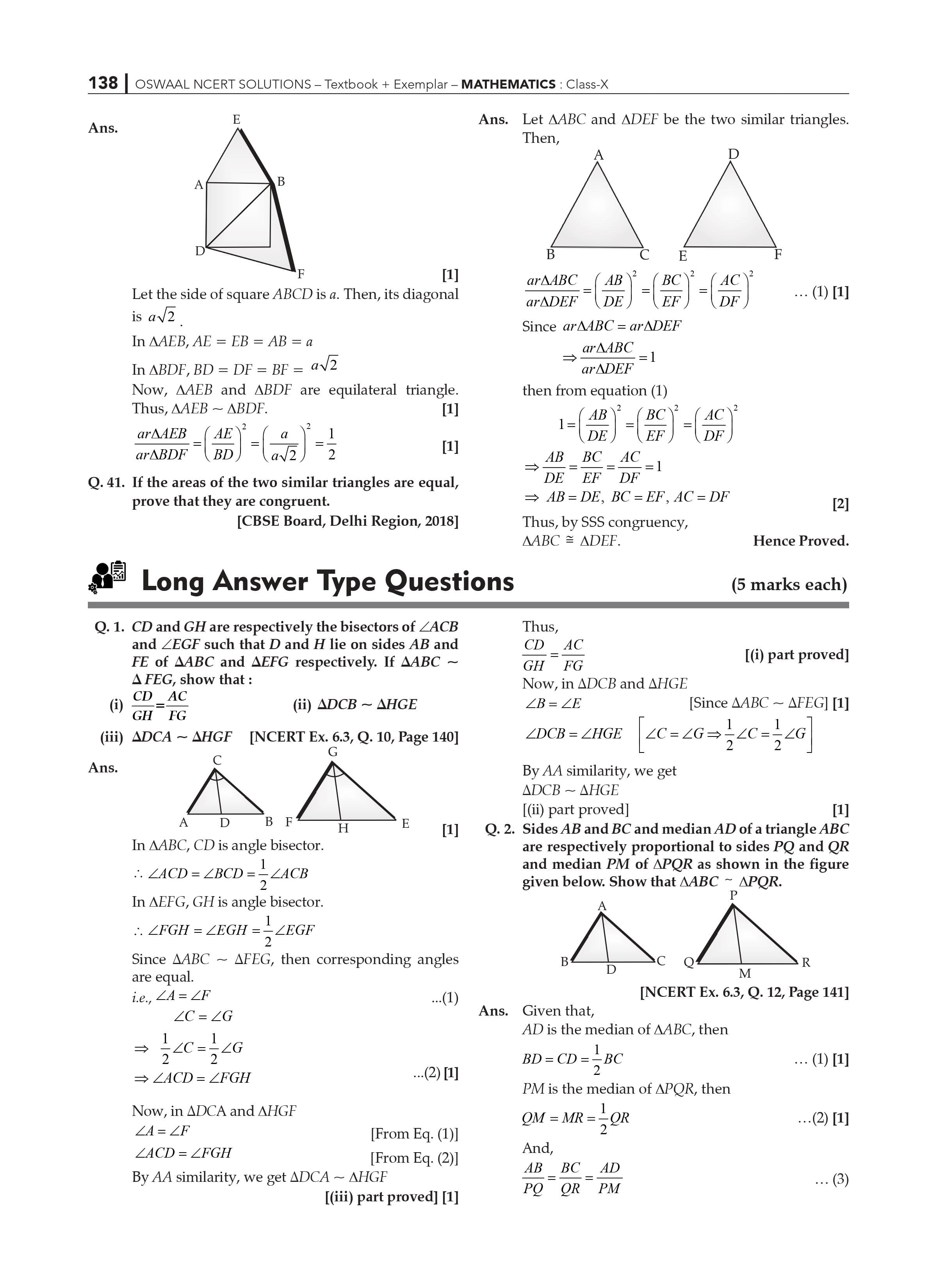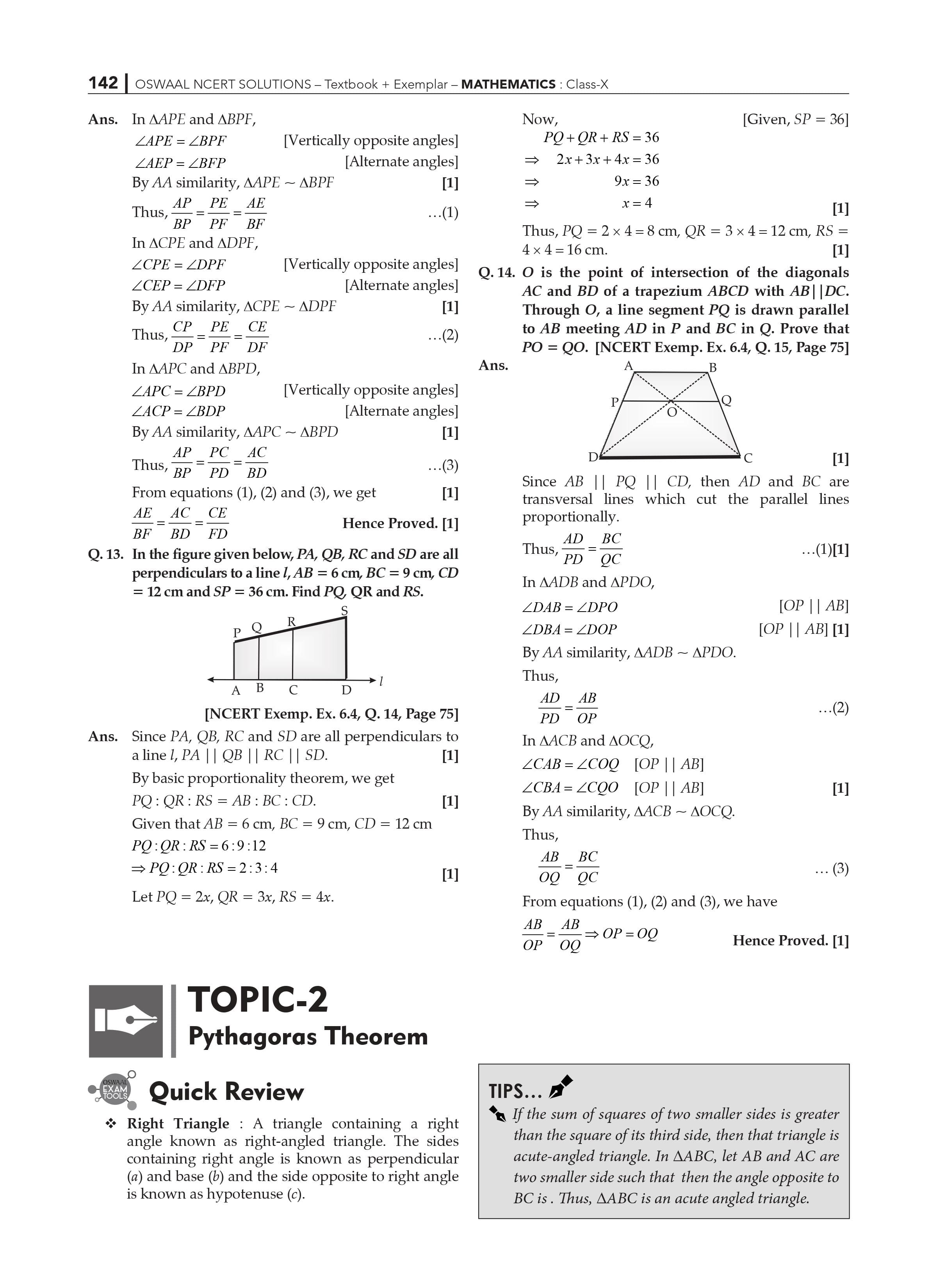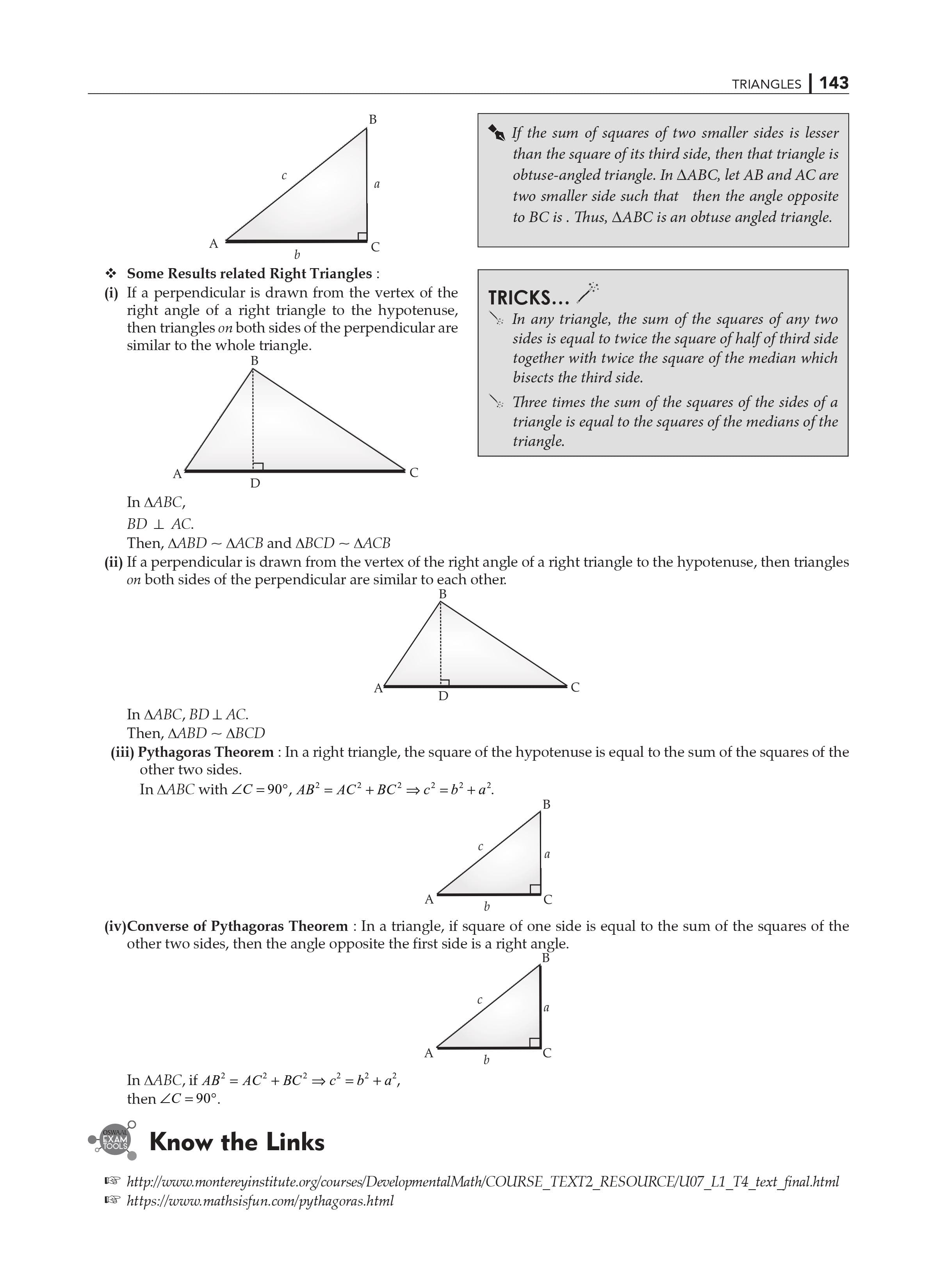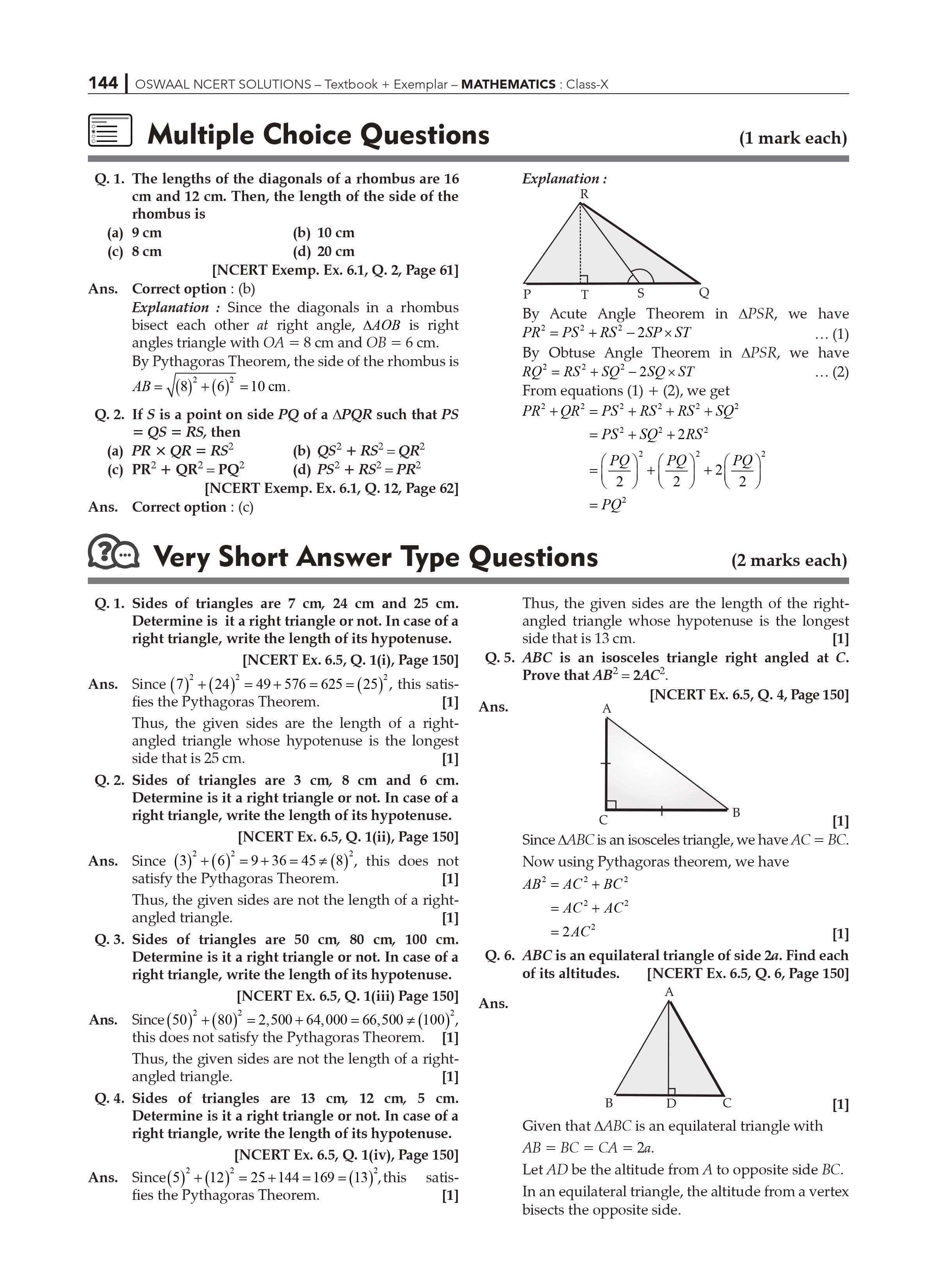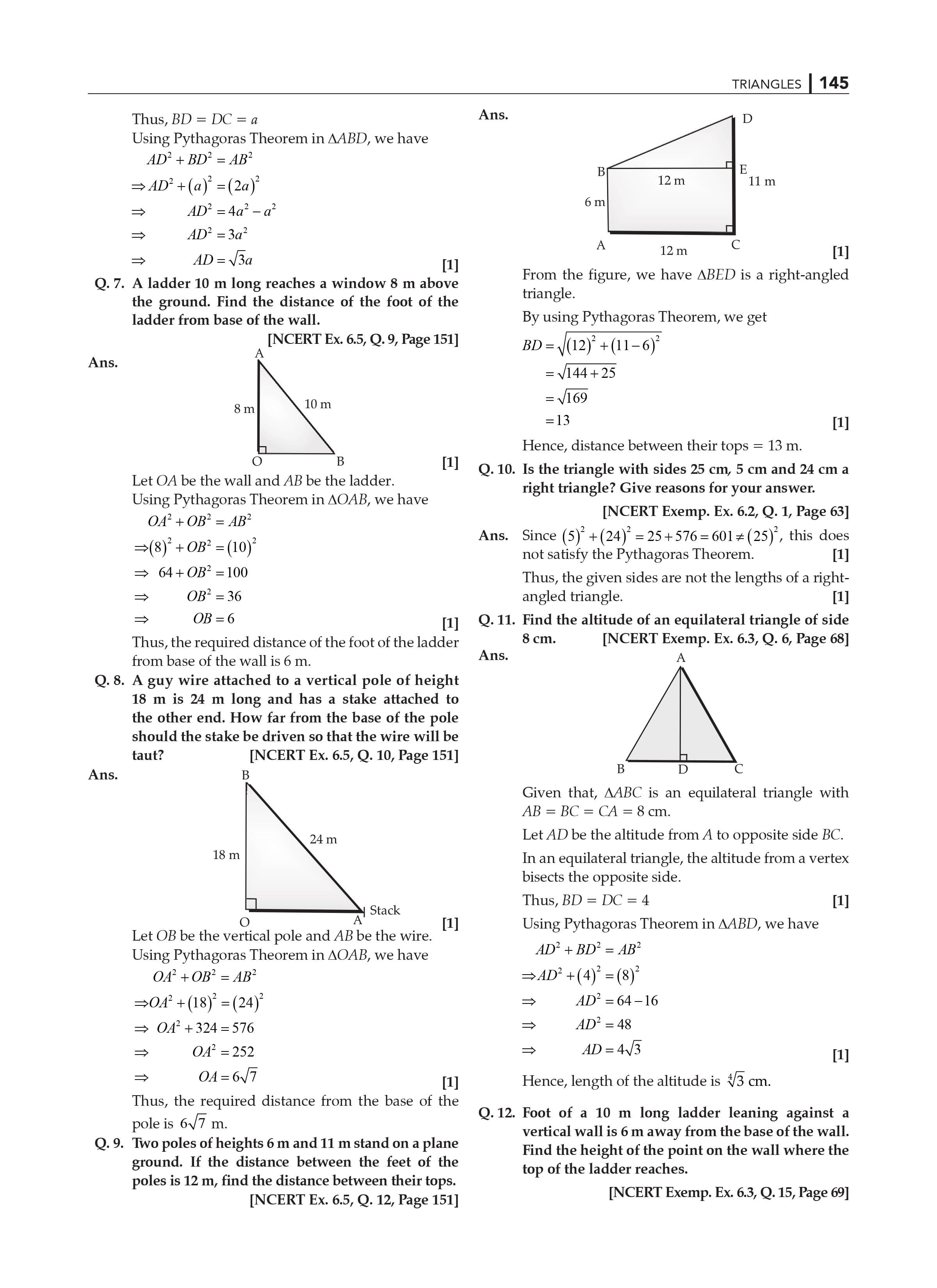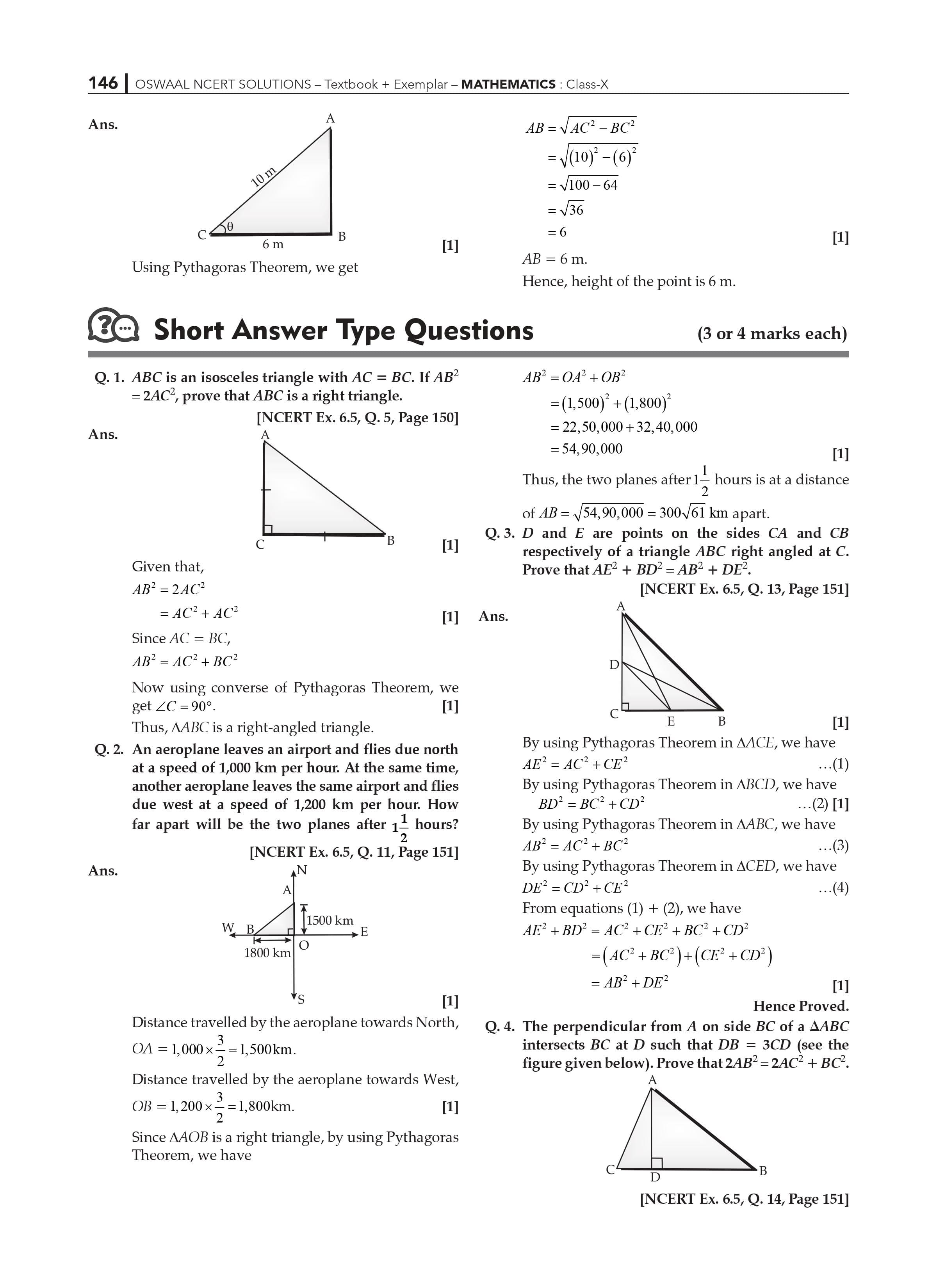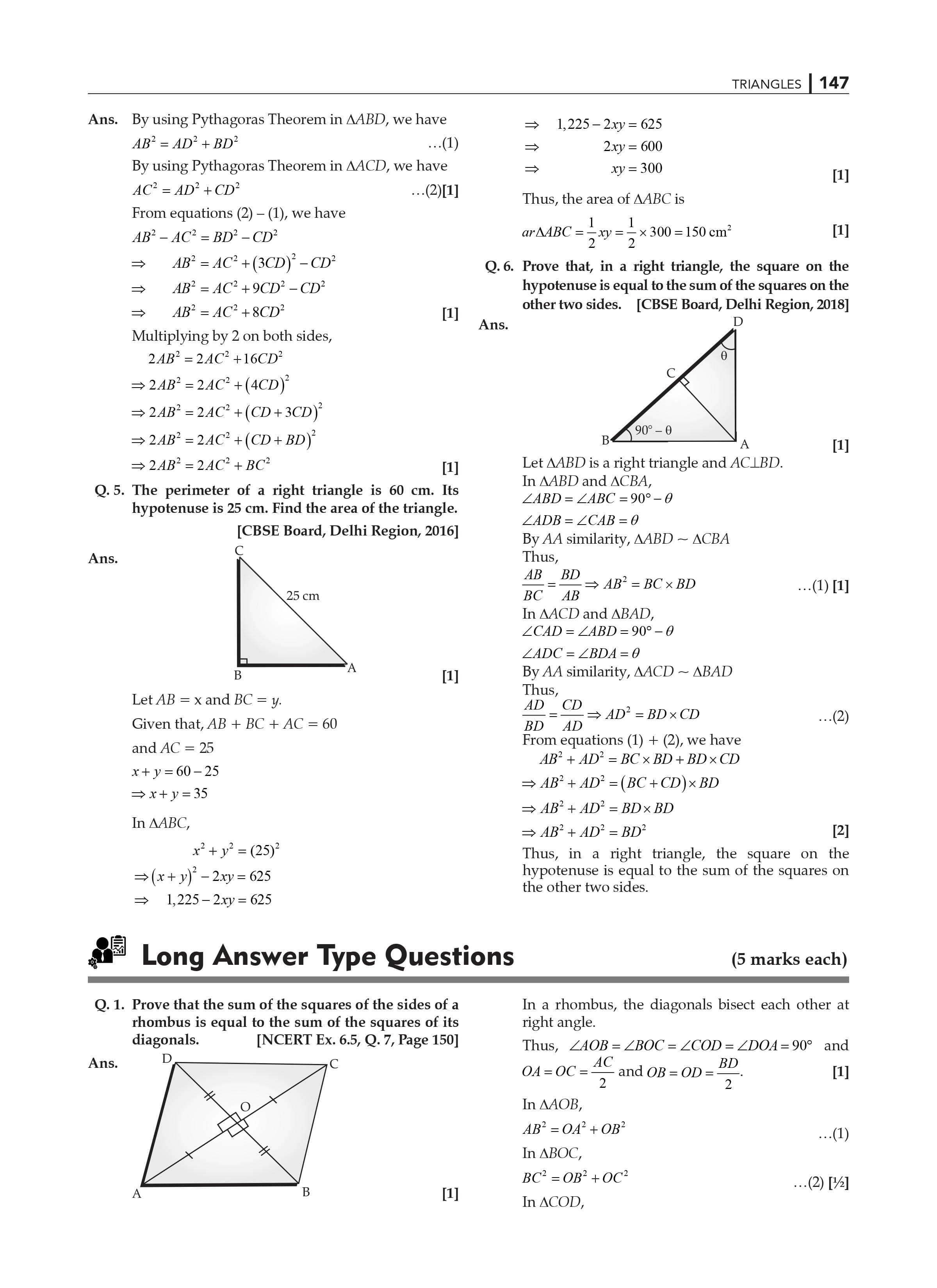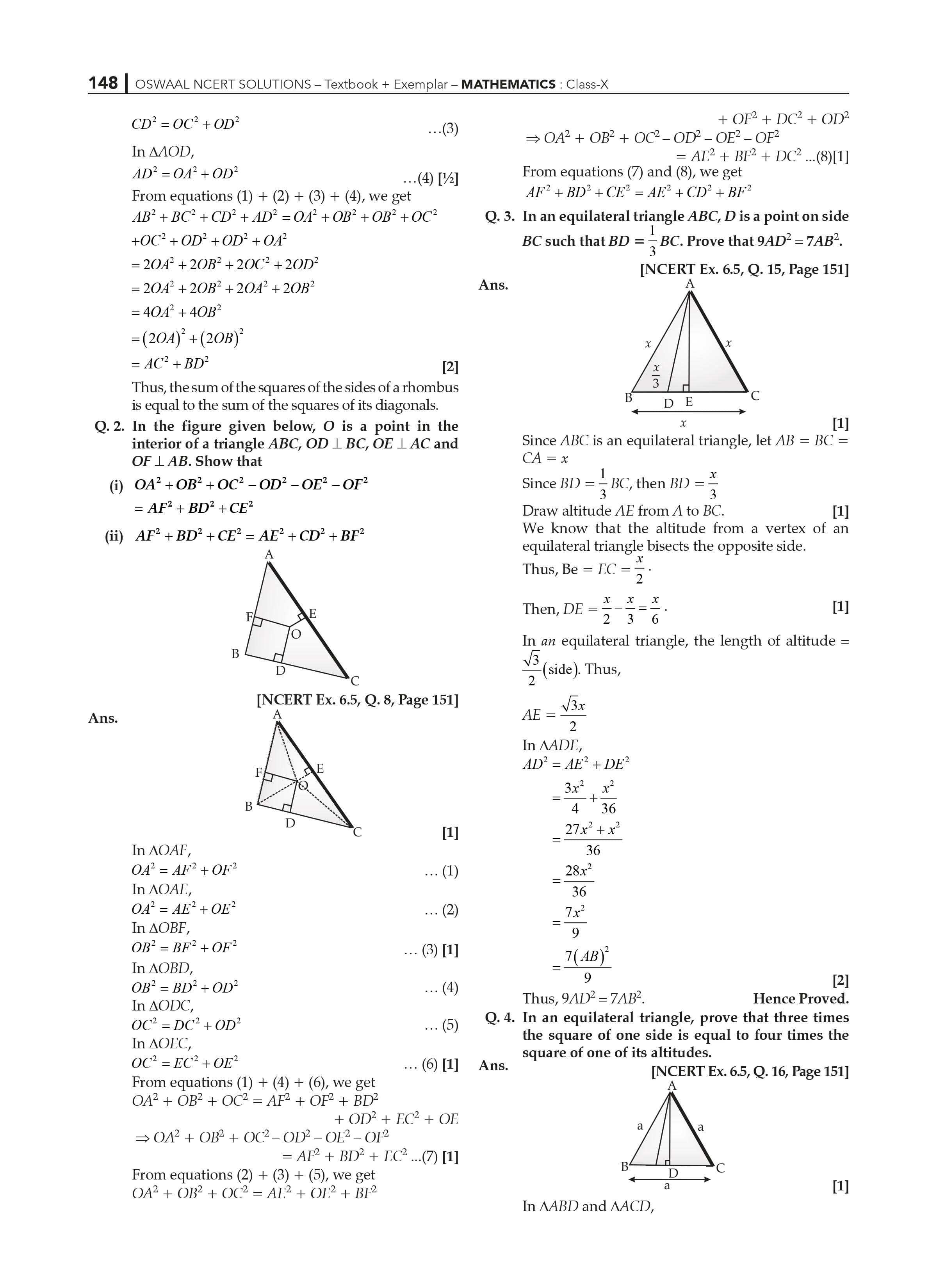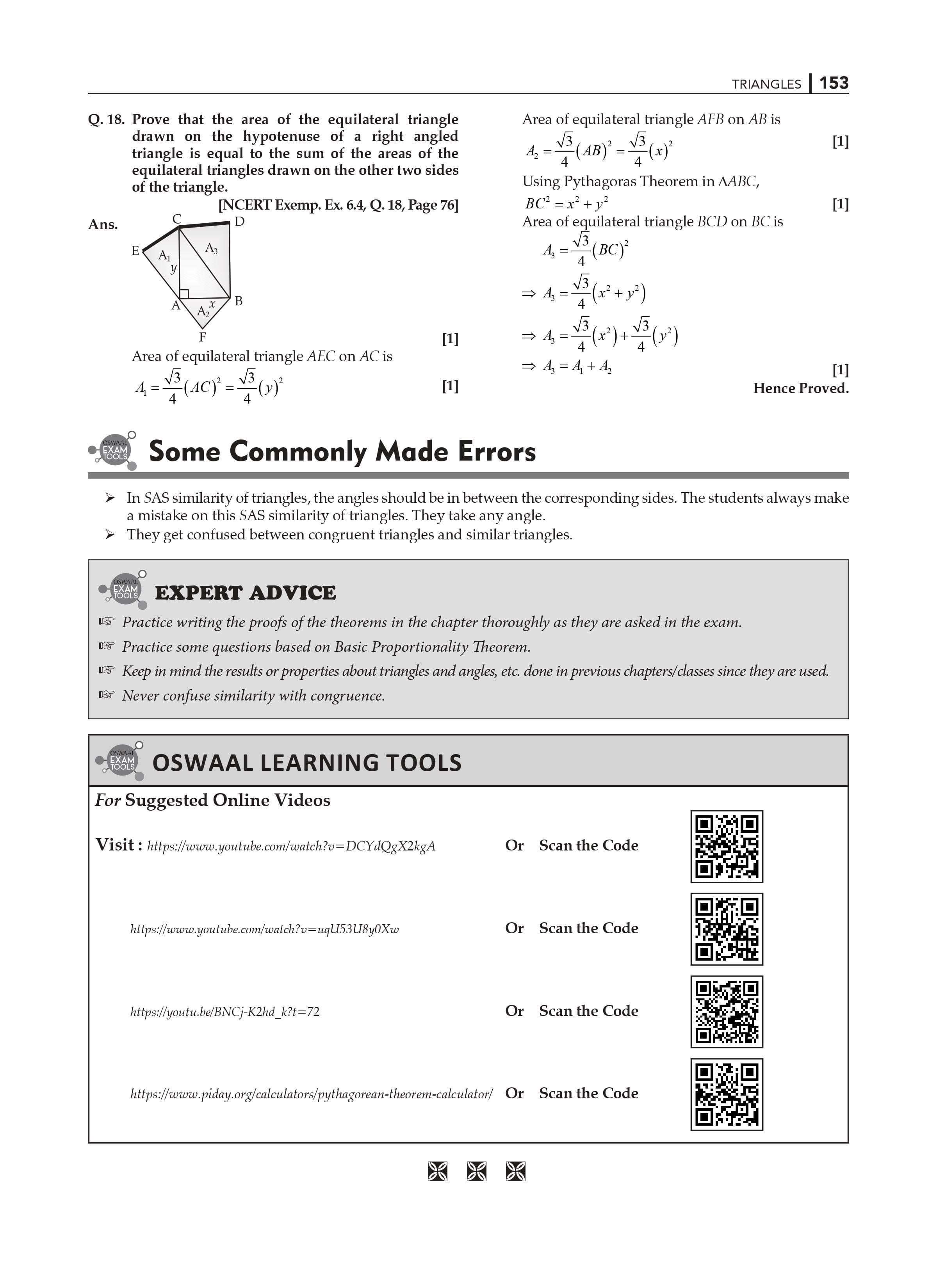Extra Information: NCERT Exemplar for Class 10 Maths Chapter 6 CBSE Notes for Class 10 Maths Chapter 6

1. If two triangles have their three side is equal is these are congruent?

Yes, it is congruent by SSS criteria.

1. If two triangles have their three angles is equal, is they are congruent?

Yes, it is congruent by AAA criteria.

If two figures have a same size and same shape than these two figures are congruent.

Similar figures are two figures that have the same shape but are not always the same size.

Congruent figures are all similar, but not all comparable forms are congruent.

The two polygon has same number of sides.

Their respective angles are the same, and their corresponding sides are proportional.

The answers to all of the questions asked in the NCERT textbook are provided in NCERT Solutions, which can assist students in practicing and learning each concept. If you want to score high in your math subject then I recommend that please do all the NCERT questions carefully with the help of our CBSE Books Class 10. This website gives you all solution of all NCERT textbooks and examples with very good concept.